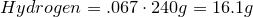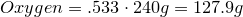# Homework 62

$$\newcommand{\vecs}{\overset { \rightharpoonup} {\mathbf{#1}} }$$ $$\newcommand{\vecd}{\overset{-\!-\!\rightharpoonup}{\vphantom{a}\smash {#1}}}$$$$\newcommand{\id}{\mathrm{id}}$$ $$\newcommand{\Span}{\mathrm{span}}$$ $$\newcommand{\kernel}{\mathrm{null}\,}$$ $$\newcommand{\range}{\mathrm{range}\,}$$ $$\newcommand{\RealPart}{\mathrm{Re}}$$ $$\newcommand{\ImaginaryPart}{\mathrm{Im}}$$ $$\newcommand{\Argument}{\mathrm{Arg}}$$ $$\newcommand{\norm}{\| #1 \|}$$ $$\newcommand{\inner}{\langle #1, #2 \rangle}$$ $$\newcommand{\Span}{\mathrm{span}}$$ $$\newcommand{\id}{\mathrm{id}}$$ $$\newcommand{\Span}{\mathrm{span}}$$ $$\newcommand{\kernel}{\mathrm{null}\,}$$ $$\newcommand{\range}{\mathrm{range}\,}$$ $$\newcommand{\RealPart}{\mathrm{Re}}$$ $$\newcommand{\ImaginaryPart}{\mathrm{Im}}$$ $$\newcommand{\Argument}{\mathrm{Arg}}$$ $$\newcommand{\norm}{\| #1 \|}$$ $$\newcommand{\inner}{\langle #1, #2 \rangle}$$ $$\newcommand{\Span}{\mathrm{span}}$$

## Q 5.105

Write the empirical formula and molecular formula for the compound with the given percent composition and molecular weight.

• 40.0% Carbon
• 6.70% Hydrogen
• 53.3% Oxygen

Molecular Weight ≈ 240 g/mol

SOLUTION

Strategy:

1. Calculate amount of grams of each element we have.
2. Convert amount of grams into number of moles of each element.
3. Obtain molecular formula.
4. Find the common factor among the number of atoms each elements has, then obtain the empirical formula.

Solution:

1. Calculate amount of grams in each element:2. Convert amount of grams into number of moles of each elements:

Carbon-Hydrogen-Oxygen-3. Obtain molecular formula:4. Find common factor and obtain empirical formula:

Common factor is 8

Empirical formula is## Q 4.88

Based on the given Ionization energies, how many valence electrons does the following atom have?

IE1=1402.3 kJ/mol IE2=2856.0 kJ/mol

IE3=4578.1 kJ/mol IE4=7475.0 kJ/mol

IE5=9444.9 kJ/mol IE6=53266.6 kJ/mol

IE7=64360.0 kJ/mol

Solution

We know that ionization energy is much larger for inner orbitals than outer orbitals. So by looking at the ionization energies given, we can see that the 6th Ionization energy is much larger than the 5th. This means that the 5th electron is the last electron in the outer shell, which means this atom has 5 valence electrons.

Homework 62 is shared under a not declared license and was authored, remixed, and/or curated by LibreTexts.